# Ball game

Richard, Denis and Denise together scored 932 goals. Denis scored 4 goals over Denise but Denis scored 24 goals less than Richard. Determine the number of goals for each player.

Correct result:

d =  304
r =  328
s =  300

#### Solution:

r+d+s = 932
d = 4 + s
d = r - 24

d+r+s = 932
d-s = 4
d-r = -24

d = 304
r = 328
s = 300

Our linear equations calculator calculates it.We would be pleased if you find an error in the word problem, spelling mistakes, or inaccuracies and send it to us. Thank you!Tips to related online calculators
Do you have a linear equation or system of equations and looking for its solution? Or do you have quadratic equation?

## Next similar math problems:

• Intercept with axisF(x)=log(x+4)-2, what is the x intercept
• Inverse matrix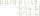Find out inverse by Gauss elimination or by reduction method. A=[2/3. 1 -3. 1/3]
• Null points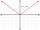Calculate the roots of the equation: ?
• Linsys2Solve two equations with two unknowns: 400x+120y=147.2 350x+200y=144
• Find d 2Find d in an A. P. whose 5th term is 18 and 39th term is 120.
• Solve 3Solve quadratic equation: (6n+1) (4n-1) = 3n2
• LegsCancer has 5 pairs of legs. The insect has 6 legs. 60 animals have a total of 500 legs. How much more are cancers than insects?
• Men, women and childrenOn the trip went men, women and children in the ratio 2:3:5 by bus. Children pay 60 crowns and adults 150. How many women were on the bus when a bus was paid 4,200 crowns?
• Linear imaginary equationGiven that ? "this is z star" Find the value of the complex number z.
• Geometric sequence 5About members of geometric sequence we know: ? ? Calculate a1 (first member) and q (common ratio or q-coefficient)
• Guppies for sale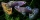Paul had a bowl of guppies for sale. Four customers were milling around the store. 1. Rod told paul - I'll take half the guppies in the bowl, plus had a guppy. 2. Heather said - I'll take half of what you have, plus half a guppy. The third customer, Nancy
• ChildrenThe group has 42 children. There are 4 more boys than girls. How many boys and girls are in the group?
• Elimination methodSolve system of linear equations by elimination method: 5/2x + 3/5y= 4/15 1/2x + 2/5y= 2/15
• AP - basicsDetermine first member and differentiate of the the following sequence: a3-a5=24 a4-2a5=61
• Gasoline canisters35 liters of gasoline is to be divided into 4 canisters so that in the third canister will have 5 liters less than in the first canister, the fourth canister 10 liters more than the third canister and the second canister half of that in the first canister
• Warehouses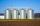In the three warehouses, a total of 70 tons of grain was stored. In the second warehouse was stored 8.5t less and in the third 3.5t more than in the first. How many tons of grain was stored in each warehouse?
• Stones 3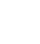Simiyu and Nasike each collected a number of stones in an arithmetic lesson. If Simiyu gave Nasike 5 stones, Nasike would have twice as many stones as Simiyu. If initially, Simiyu had five stones less than Nasike how many stones did each have?